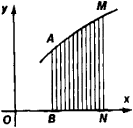# Variables and Constants

The following article is from The Great Soviet Encyclopedia (1979). It might be outdated or ideologically biased.

## Variables and Constants

quantities that, respectively, take on different values and maintain the same value in a problem under study. For example, in the study of a falling body, the distance of the body from the earth and the body’s speed are variables, and the body’s acceleration—if air resistance is neglected—is a constant.

Early mathematics regarded all quantities studied by it as constants. The concept of a variable arose in mathematics in the 17th century in response to the needs of natural science, which had begun to devote great attention to the study of motion—that is, processes—as well as states. The concept was incompatible with the forms developed by the mathematicians of antiquity and the Middle Ages and required new forms for its expression. Such new forms were provided by the literal algebra and analytic geometry of R. Descartes. Variables found symbolic expression in the letters of Descartes’s algebra, which were capable of assuming arbitrary numerical values. “Descartes’s variable quantity was a turning point in mathematics. It brought motion and, thereby, dialectics into mathematics. As a result, differential and integral calculus quickly became necessary...” (F. Engels, see K. Marx and F. Engels, Soch., 2nd ed., vol. 20, p. 573).

From this period through the mid-19th century, mechanistic conceptions of variables predominated. They were most clearly expressed by I. Newton, who called variables “fluents”—that is, flowing quantities—and regarded them “not as consisting of very small parts but as described by a continuous motion” (Matematicheskie raboty, Moscow, 1937, p. 167). These conceptions proved to be very fruitful and, in particular, enabled Newton to find the areas of curvilinear figures by a completely new approach. Newton was the first to view the area under a curve— for example, the area of ABNM in Figure 1—not as a constant that was computable by summing infinitesimal parts but as a variable produced by the motion of the ordinate NM of the curve. By establishing that the rate of change of the area is proportional to NM, he reduced the problem of computing areas to the problem of determining a variable from its known rate of change. The legitimacy of introducing the concept of rate of change into mathematics was substantiated in the early 19th century by the theory of limits, which gave a precise definition of rates of change as derivatives.Figure 1

In the course of the 19th century, however, the limitedness of the above view of variables gradually became clear. Mathematical analysis became more and more a general theory of functions, and the theory could not develop without a precise analysis of the nature and extent of its basic concepts. Moreover, the concept of continuous function proved in reality to be considerably more complicated than the obvious ideas that led to it. Continuous functions were discovered that did not have a derivative at any point; to understand such a function as a result of motion would mean to admit the possibility of motion without any speed at any moment. The study of discontinuous functions acquired increasing importance, as did that of functions defined on sets of considerably more complicated structure than an interval or union of intervals. The Newtonian interpretation of variables became inadequate and in many cases even useless.

On the other hand, mathematics began considering as variables not only quantities but also more and more diverse and broad classes of objects. Set theory, topology, and mathematical logic all developed on this basis in the second half of the 19th century and in the 20th century. The extent to which the concept of variable has been broadened in the 20th century is so great that mathematical logic deals not only with variables that run through arbitrary sets of objects but also with variables whose values may be, for example, statements or predicates (relations between objects).

The Great Soviet Encyclopedia, 3rd Edition (1970-1979). © 2010 The Gale Group, Inc. All rights reserved.
Site: Follow: Share:
Open / Close